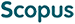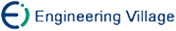吴高巍, 陶 卿, 王 珏. 基于后验概率的支持向量机[J]. 计算机研究与发展, 2005, 42(2): 196-202.
 引用本文: 吴高巍, 陶 卿, 王 珏. 基于后验概率的支持向量机[J]. 计算机研究与发展, 2005, 42(2): 196-202.Wu Gaowei, Tao Qing, Wang Jue. Support Vector Machines Based on Posteriori Probability[J]. Journal of Computer Research and Development, 2005, 42(2): 196-202.
 Citation: Wu Gaowei, Tao Qing, Wang Jue. Support Vector Machines Based on Posteriori Probability[J]. Journal of Computer Research and Development, 2005, 42(2): 196-202.## Support Vector Machines Based on Posteriori Probability

• 摘要: 在支持向量机(support vector machines,SVM)中，训练样本总是具有明确的类别信息，而对于一些不确定性问题并不恰当.受贝叶斯决策规则的启发，利用样本的后验概率来表示这种不确定性.将贝叶斯决策规则与SVM相结合，建立后验概率支持向量机(posteriori probability support vector machine，PPSVM)的体系框架.并详细讨论线性可分性、间隔、最优超平面以及软间隔算法，得到了一个新的优化问题，同时给出了一个支持向量的新定义.实际上， 后验概率支持向量机是建立于统计学习理论(statistical learning theory)基础之上，是标准SVM的扩展.针对数据，还提出了一个确定后验概率的经验性方法.实验也证明了后验概率支持向量机的合理性、有效性.

Abstract: To solve uncertain classification problem, an SVM (support vector machine) is trained to behave like a Bayesian optimal classifier based on the training data. The idea is to weigh each unbalanced training sample by a posteriori probability. A whole framework of posteriori probability support vector machine (PPSVM) is presented and SVM is reformulated into PPSVM. The linear separability, margin, optimal hyperplane and soft margin algorithms are discussed. A new optimization problem is obtained and a new definition of support vector is given. In fact, PPSVM is motivated by statistical learning theory and is an extension of regular SVM. An empirical method is also proposed for determining the posteriori probability. Two artificial examples show that PPSVM formulation is reasonable if the class-conditional probability is known, and some real experiments demonstrate that the weighted data cases by some empirical methods can produce better results than regular SVM./下载:  全尺寸图片 幻灯片
• 分享
• 用微信扫码二维码

分享至好友和朋友圈